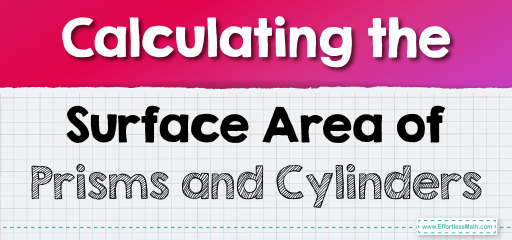# Calculating the Surface Area of Prisms and Cylinders

From the skyscrapers that pierce the skyline to the soda cans in our fridges, prisms and cylinders are foundational shapes in our daily lives. But while their outward appearance may seem straightforward, there's an underlying mathematical beauty in determining just how much space they cover on the outside. In this guide, we'll dive deep into the methodologies to calculate the surface area of prisms and cylinders, shedding light on the formulas and techniques that bring these 3D figures to life. Join us in this geometric journey to explore the surfaces of the world around us!## Step-by-step Guide: Surface Area of Prisms and Cylinders

Prism: A prism is a polyhedron with two parallel and congruent bases. The sides (lateral faces) are parallelograms. The surface area is the total area covering the prism.

Surface Area $$SA = 2 \times \text{Base Area} + \text{Perimeter of Base} \times \text{Height}$$

Cylinder: A cylinder has two congruent, parallel bases and a curved surface.

Surface Area $$SA = 2\pi r^2 + 2\pi rh$$

Where:
$$r =$$ radius of the base
$$h =$$ height of the cylinder

### Examples

Example 1:
Find the surface area of a rectangular prism with a length of $$7 \text{ cm}$$, width of $$5 \text{ cm}$$, and height of $$10 \text{ cm}$$.

Solution:
Base Area $$= 7 \text{ cm} \times 5 \text{ cm} = 35 \text{ cm}^2$$

Perimeter of Base $$= 2(7 \text{ cm} + 5 \text{ cm}) = 24 \text{ cm}$$

$$SA = (2 \times 35 \text{ cm}^2) + (24 \text{ cm} \times 10 \text{ cm}) = 310 \text{ cm}^2$$

Example 2:
Calculate the surface area of a cylinder with a radius of $$4 \text{ cm}$$ and a height of $$9 \text{ cm}$$.

Solution:
$$SA = 2\pi (4 \text{ cm})^2 + 2\pi (4 \text{ cm})(9 \text{ cm}) = 326.56 \text{ cm}^2$$

### Practice Questions:

1. Determine the surface area of a rectangular prism with dimensions $$6 \text{ cm} \times 5 \text{ cm} \times 8 \text{ cm}$$.
2. What is the surface area of a cylinder with a radius of $$5 \text{ cm}$$ and a height of $$10 \text{ cm}$$?

1. $$236 \text{ cm}^2$$
2. $$471 \text{ cm}^2$$

### What people say about "Calculating the Surface Area of Prisms and Cylinders - Effortless Math: We Help Students Learn to LOVE Mathematics"?

No one replied yet.

X
30% OFF

Limited time only!

Save Over 30%

SAVE $5 It was$16.99 now it is \$11.99